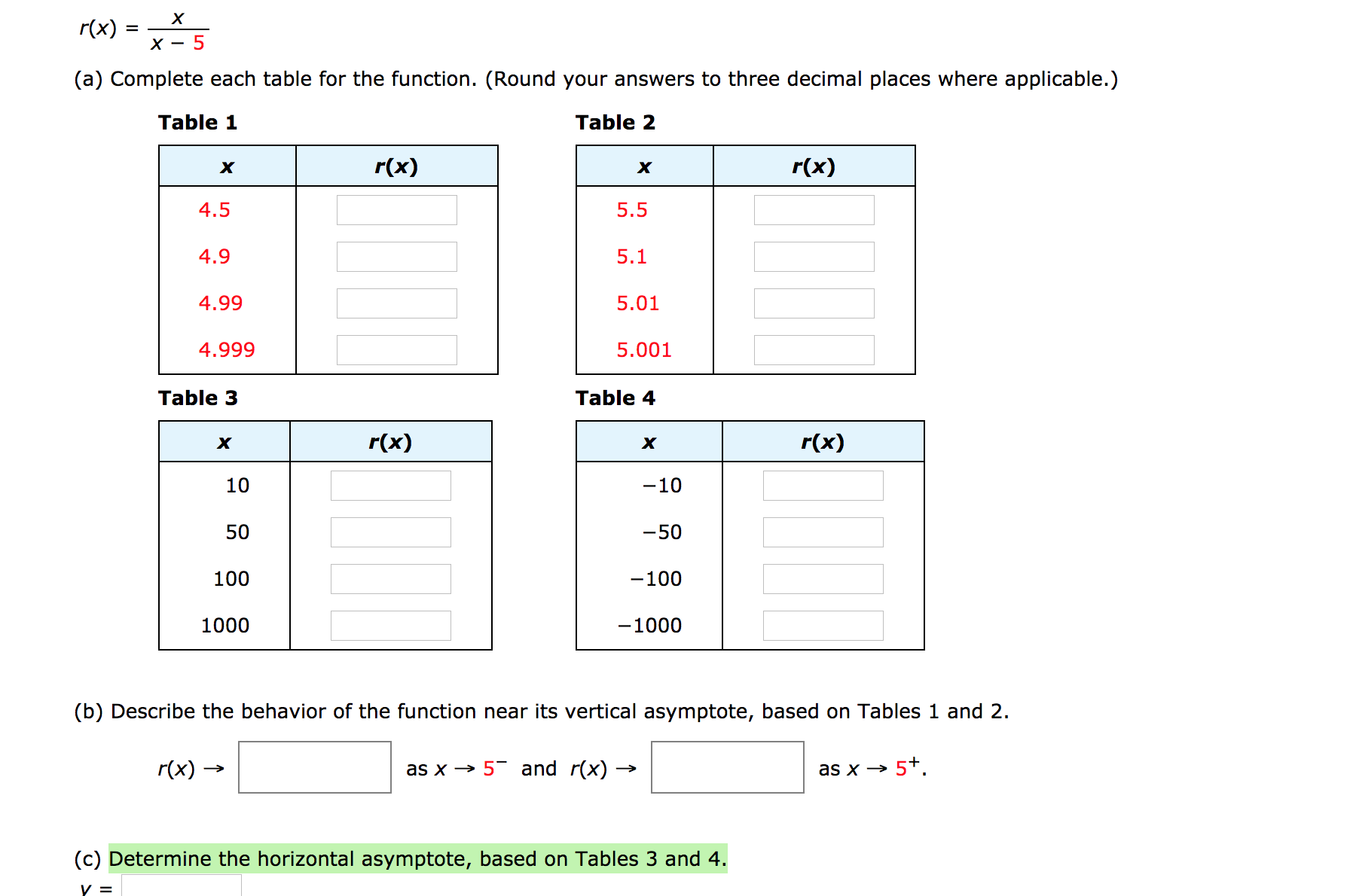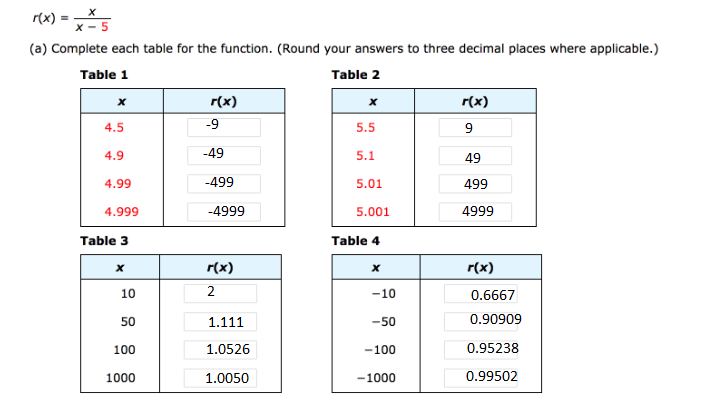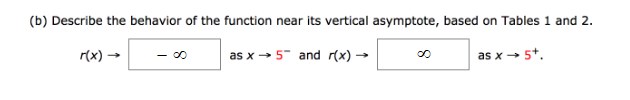# хr(x)(a) Complete each table for the function. (Round your answers to three decimal places where applicable.)Table 1Table 2r(x)r(x)хх4.55.54.95.14.995.014.9995.001Table 4Table 3r(x)r(x)хх10-1050-50100-100-10001000(b) Describe the behavior of the function near its vertical asymptote, based on Tables 1 and 2.r(x) –as x → 5 and r(x) -as x → 5+.(c) Determine the horizontal asymptote, based on Tables 3 and 4.

Question
47 views

A rational function is given

r(x)= x/x-5

a.) complete each table for the function. (Round your answers to three decimal places where applicable.)

(b) Describe the behavior of the function near its vertical asymptote, based on Tables 1 and 2.

c.) Determine the horizontal asymptote, based on Tables 3 and 4.help_outlineImage Transcriptioncloseх r(x) (a) Complete each table for the function. (Round your answers to three decimal places where applicable.) Table 1 Table 2 r(x) r(x) х х 4.5 5.5 4.9 5.1 4.99 5.01 4.999 5.001 Table 4 Table 3 r(x) r(x) х х 10 -10 50 -50 100 -100 -1000 1000 (b) Describe the behavior of the function near its vertical asymptote, based on Tables 1 and 2. r(x) – as x → 5 and r(x) - as x → 5+. (c) Determine the horizontal asymptote, based on Tables 3 and 4. fullscreen
check_circle

(a)(b)...

### Want to see the full answer?

See Solution

#### Want to see this answer and more?

Solutions are written by subject experts who are available 24/7. Questions are typically answered within 1 hour.*

See Solution
*Response times may vary by subject and question.
Tagged in